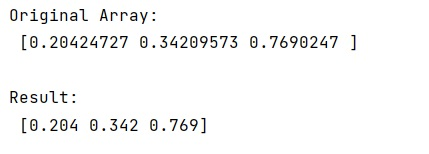# How to print numpy array with 3 decimal places?

Learn, how to print numpy array with 3 decimal places in Python?
Submitted by Pranit Sharma, on February 17, 2023

NumPy is an abbreviated form of Numerical Python. It is used for different types of scientific operations in python. Numpy is a vast library in python which is used for almost every kind of scientific or mathematical operation. It is itself an array which is a collection of various methods and functions for processing the arrays.

## Printing numpy array with 3 decimal places

Suppose that we are given a numpy array that contains long decimal values and we need to print its values up to 3 decimal places.

For this purpose, numpy provides us a simple method called set_printoptions() which we can use and define a lambda function inside it to set the decimal places to 3 points using "{0:0.3f}".format().

This will set numpy to use this lambda function for formatting every float it prints out.

There are certainly other data types that we can define for formatting:

• 'timedelta' : a `numpy.timedelta64`
• 'datetime' : a `numpy.datetime64`
• 'float'
• 'longfloat' : 128-bit floats
• 'complexfloat'
• 'longcomplexfloat' : composed of two 128-bit floats
• 'numpy_str' : types `numpy.string_` and `numpy.unicode_`
• 'str' : all other strings

Let us understand with the help of an example,

## Python code to print numpy array with 3 decimal places

```# Import numpy
import numpy as np

# Creating a numpy array
arr = np.array([0.20424727482457, 0.342095729840246, 0.7690247024585])

# Display original array
print("Original Array:\n",arr,"\n")

# Printing elements of array up to 3 decimal places
np.set_printoptions(formatter={'float': lambda x: "{0:0.3f}".format(x)})

# Display Result
print("Result:\n",arr)
```

Output: